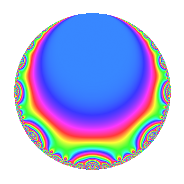# Properties

 Label 8036.2.a.gLevel 8036 Weight 2 Character orbit 8036.a Self dual Yes Analytic conductor 64.168 Analytic rank 0 Dimension 2 CM No Inner twists 1

# Related objects

## Newspace parameters

 Level: $$N$$ = $$8036 = 2^{2} \cdot 7^{2} \cdot 41$$ Weight: $$k$$ = $$2$$ Character orbit: $$[\chi]$$ = 8036.a (trivial)

## Newform invariants

 Self dual: Yes Analytic conductor: $$64.1677830643$$ Analytic rank: $$0$$ Dimension: $$2$$ Coefficient field: $$\Q(\sqrt{13})$$ Coefficient ring: $$\Z[a_1, a_2, a_3]$$ Coefficient ring index: $$1$$ Fricke sign: $$-1$$ Sato-Tate group: $\mathrm{SU}(2)$

## $q$-expansion

Coefficients of the $$q$$-expansion are expressed in terms of $$\beta = \frac{1}{2}(1 + \sqrt{13})$$. We also show the integral $$q$$-expansion of the trace form.

 $$f(q)$$ $$=$$ $$q + ( 1 + \beta ) q^{3} + ( 1 + \beta ) q^{5} + ( 1 + 3 \beta ) q^{9} +O(q^{10})$$ $$q + ( 1 + \beta ) q^{3} + ( 1 + \beta ) q^{5} + ( 1 + 3 \beta ) q^{9} + q^{11} + 5 q^{13} + ( 4 + 3 \beta ) q^{15} + ( 3 - 4 \beta ) q^{17} + ( 3 - 3 \beta ) q^{19} + ( -1 - \beta ) q^{23} + ( -1 + 3 \beta ) q^{25} + ( 7 + 4 \beta ) q^{27} + ( 8 + \beta ) q^{29} + ( -7 + 3 \beta ) q^{31} + ( 1 + \beta ) q^{33} + ( 4 - 2 \beta ) q^{37} + ( 5 + 5 \beta ) q^{39} + q^{41} + ( -7 - 2 \beta ) q^{43} + ( 10 + 7 \beta ) q^{45} + ( 10 - 2 \beta ) q^{47} + ( -9 - 5 \beta ) q^{51} + ( -5 + \beta ) q^{53} + ( 1 + \beta ) q^{55} + ( -6 - 3 \beta ) q^{57} + ( 1 - 3 \beta ) q^{59} + ( 1 + 4 \beta ) q^{61} + ( 5 + 5 \beta ) q^{65} + ( -5 + 3 \beta ) q^{67} + ( -4 - 3 \beta ) q^{69} + ( -3 - 4 \beta ) q^{71} -7 q^{73} + ( 8 + 5 \beta ) q^{75} + ( -12 - 2 \beta ) q^{79} + ( 16 + 6 \beta ) q^{81} + ( -3 + 6 \beta ) q^{83} + ( -9 - 5 \beta ) q^{85} + ( 11 + 10 \beta ) q^{87} + ( -3 + 5 \beta ) q^{89} + ( 2 - \beta ) q^{93} + ( -6 - 3 \beta ) q^{95} + ( 7 - 7 \beta ) q^{97} + ( 1 + 3 \beta ) q^{99} +O(q^{100})$$ $$\operatorname{Tr}(f)(q)$$ $$=$$ $$2q + 3q^{3} + 3q^{5} + 5q^{9} + O(q^{10})$$ $$2q + 3q^{3} + 3q^{5} + 5q^{9} + 2q^{11} + 10q^{13} + 11q^{15} + 2q^{17} + 3q^{19} - 3q^{23} + q^{25} + 18q^{27} + 17q^{29} - 11q^{31} + 3q^{33} + 6q^{37} + 15q^{39} + 2q^{41} - 16q^{43} + 27q^{45} + 18q^{47} - 23q^{51} - 9q^{53} + 3q^{55} - 15q^{57} - q^{59} + 6q^{61} + 15q^{65} - 7q^{67} - 11q^{69} - 10q^{71} - 14q^{73} + 21q^{75} - 26q^{79} + 38q^{81} - 23q^{85} + 32q^{87} - q^{89} + 3q^{93} - 15q^{95} + 7q^{97} + 5q^{99} + O(q^{100})$$

## Embeddings

For each embedding $$\iota_m$$ of the coefficient field, the values $$\iota_m(a_n)$$ are shown below.

For more information on an embedded modular form you can click on its label.

Label $$\iota_m(\nu)$$ $$a_{2}$$ $$a_{3}$$ $$a_{4}$$ $$a_{5}$$ $$a_{6}$$ $$a_{7}$$ $$a_{8}$$ $$a_{9}$$ $$a_{10}$$
1.1
 −1.30278 2.30278
0 −0.302776 0 −0.302776 0 0 0 −2.90833 0
1.2 0 3.30278 0 3.30278 0 0 0 7.90833 0
 $$n$$: e.g. 2-40 or 990-1000 Significant digits: Format: Complex embeddings Normalized embeddings Satake parameters Satake angles

## Inner twists

This newform does not admit any (nontrivial) inner twists.

## Atkin-Lehner signs

$$p$$ Sign
$$2$$ $$-1$$
$$7$$ $$-1$$
$$41$$ $$-1$$

## Hecke kernels

This newform can be constructed as the intersection of the kernels of the following linear operators acting on $$S_{2}^{\mathrm{new}}(\Gamma_0(8036))$$:

 $$T_{3}^{2} - 3 T_{3} - 1$$ $$T_{5}^{2} - 3 T_{5} - 1$$ $$T_{11} - 1$$